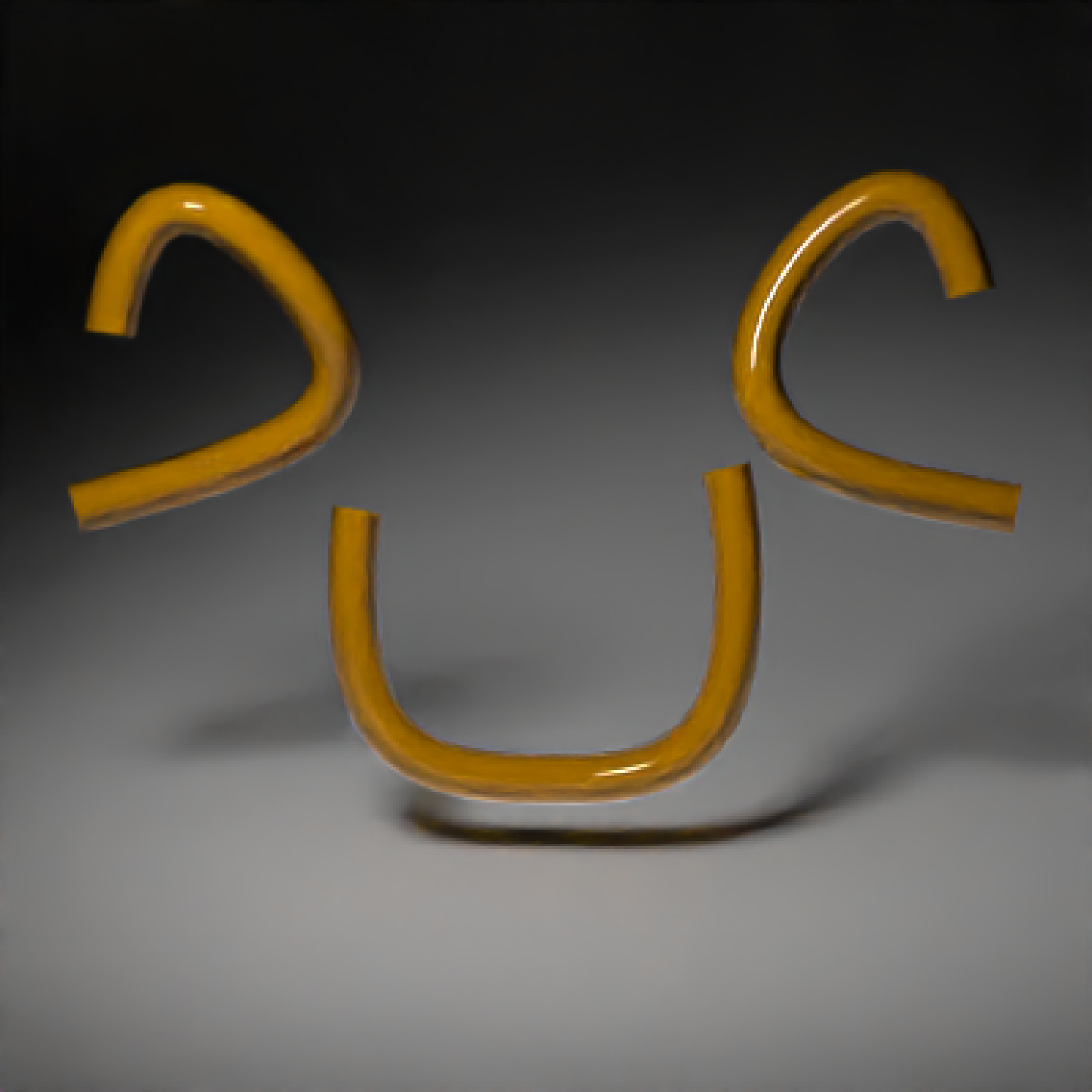Either a closed or open path made up of bezier curves that go through the specified points (with continuous first and second derivatives), or straight line segments.

path(
points,
x = 0,
y = 0,
z = 0,
closed = FALSE,
closed_smooth = TRUE,
straight = FALSE,
precomputed_control_points = FALSE,
width = 0.1,
width_end = NA,
u_min = 0,
u_max = 1,
type = "cylinder",
normal = c(0, 0, -1),
normal_end = NA,
material = diffuse(),
angle = c(0, 0, 0),
order_rotation = c(1, 2, 3),
flipped = FALSE,
scale = c(1, 1, 1)
)

## Arguments

points

Either a list of length-3 numeric vectors or 3-column matrix/data.frame specifying the x/y/z points that the path should go through.

x

Default 0. x-coordinate offset for the path.

y

Default 0. y-coordinate offset for the path.

z

Default 0. z-coordinate offset for the path.

closed

Default FALSE. If TRUE, the path will be closed by smoothly connecting the first and last points.

closed_smooth

Default TRUE. If closed = TRUE, this will ensure C2 (second derivative) continuity between the ends. If closed = FALSE, the curve will only have C1 (first derivative) continuity between the ends.

straight

Default FALSE. If TRUE, straight lines will be used to connect the points instead of bezier curves.

precomputed_control_points

Default FALSE. If TRUE, points argument will expect a list of control points calculated with the internal rayrender function rayrender:::calculate_control_points().

width

Default 0.1. Curve width.

width_end

Default NA. Width at end of path. Same as width, unless specified.

u_min

Default 0. Minimum parametric coordinate for the path.

u_max

Default 1. Maximum parametric coordinate for the path.

type

Default cylinder. Other options are flat and ribbon.

normal

Default c(0,0,-1). Orientation surface normal for the start of ribbon curves.

normal_end

Default NA. Orientation surface normal for the start of ribbon curves. If not specified, same as normal.

material

Default diffuse.The material, called from one of the material functions diffuse, metal, or dielectric.

angle

Default c(0, 0, 0). Angle of rotation around the x, y, and z axes, applied in the order specified in order_rotation.

order_rotation

Default c(1, 2, 3). The order to apply the rotations, referring to "x", "y", and "z".

flipped

Default FALSE. Whether to flip the normals.

scale

Default c(1, 1, 1). Scale transformation in the x, y, and z directions. If this is a single value, number, the object will be scaled uniformly. Note: emissive objects may not currently function correctly when scaled.

## Value

Single row of a tibble describing the cube in the scene.

## Examples

if(run_documentation()) {
#Generate a wavy line, showing the line goes through the specified points:
wave = list(c(-2,1,0),c(-1,-1,0),c(0,1,0),c(1,-1,0),c(2,1,0))
point_mat = glossy(color="green")
generate_studio(depth=-1.5) %>%
render_scene(samples=128, clamp_value=10,fov=30)
}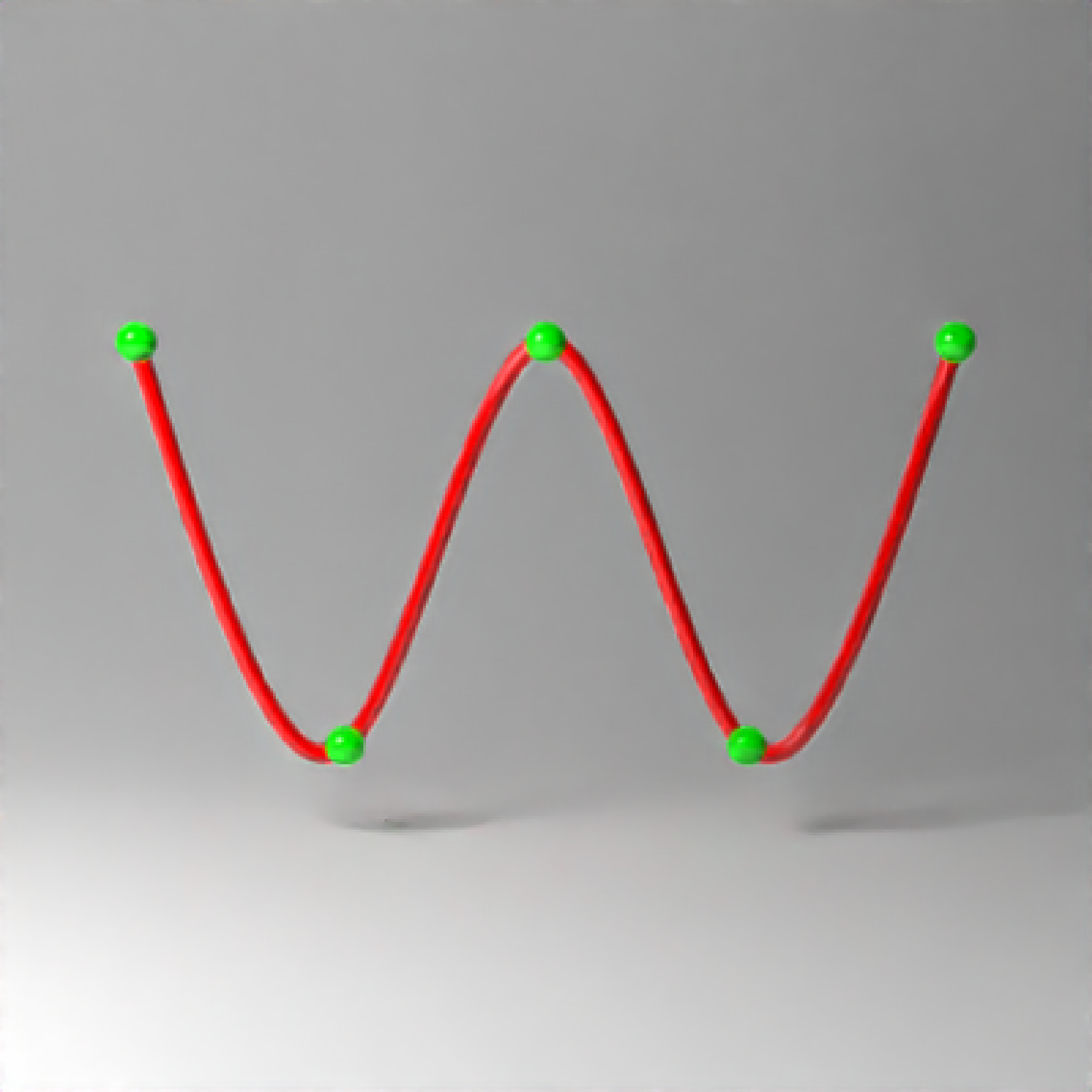if(run_documentation()) {
#Here we use straight lines by setting straight = TRUE:
generate_studio(depth=-1.5) %>%
add_object(path(points = wave,straight = TRUE, material=glossy(color="red"))) %>%
render_scene(samples=128, clamp_value=10,fov=30)
}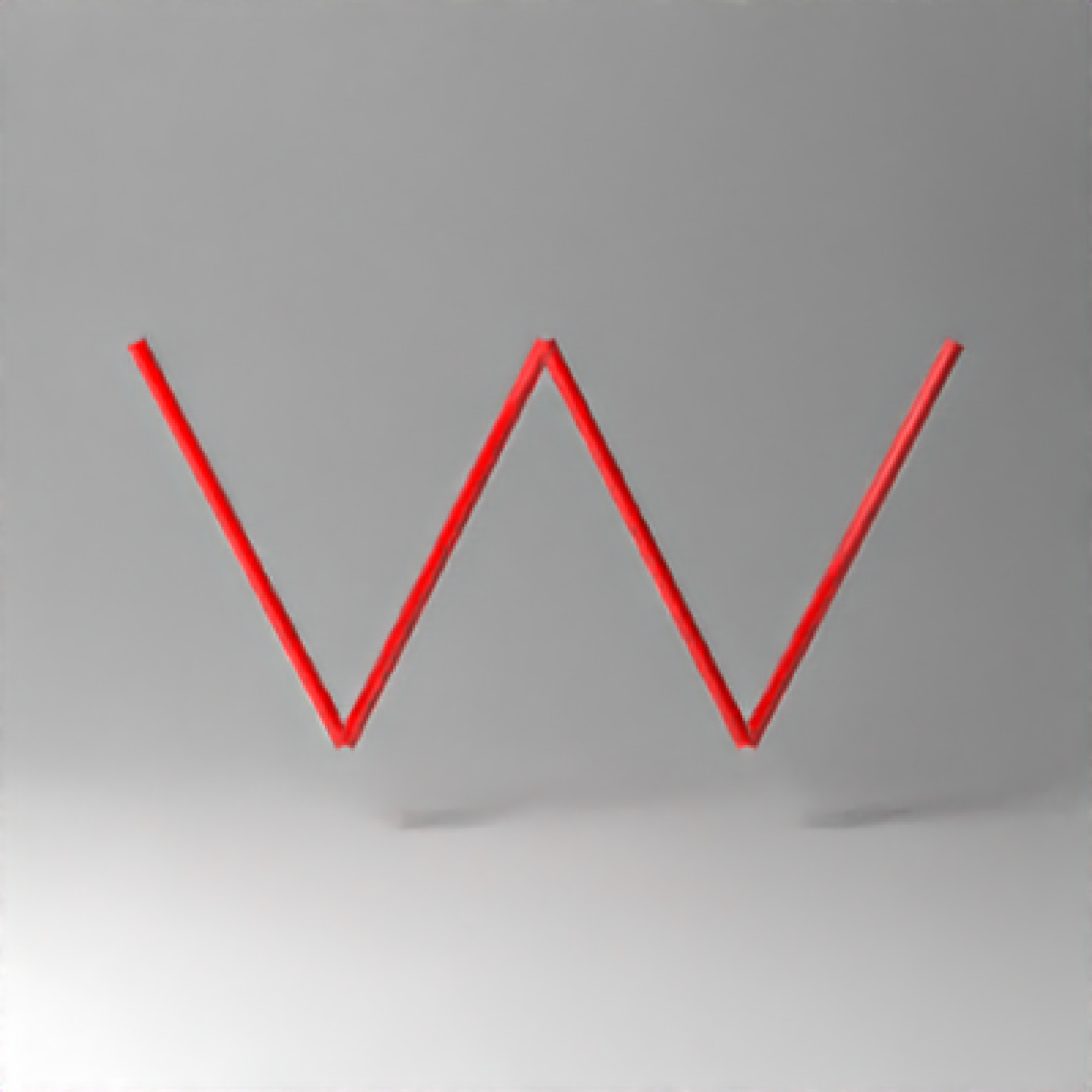if(run_documentation()) {
#We can also pass a matrix of values, specifying the x/y/z coordinates. Here,
#we'll create a random curve:
set.seed(21)
random_mat = matrix(runif(3*9)*2-1, ncol=3)
generate_studio(depth=-1.5) %>%
render_scene(samples=128, clamp_value=10)
}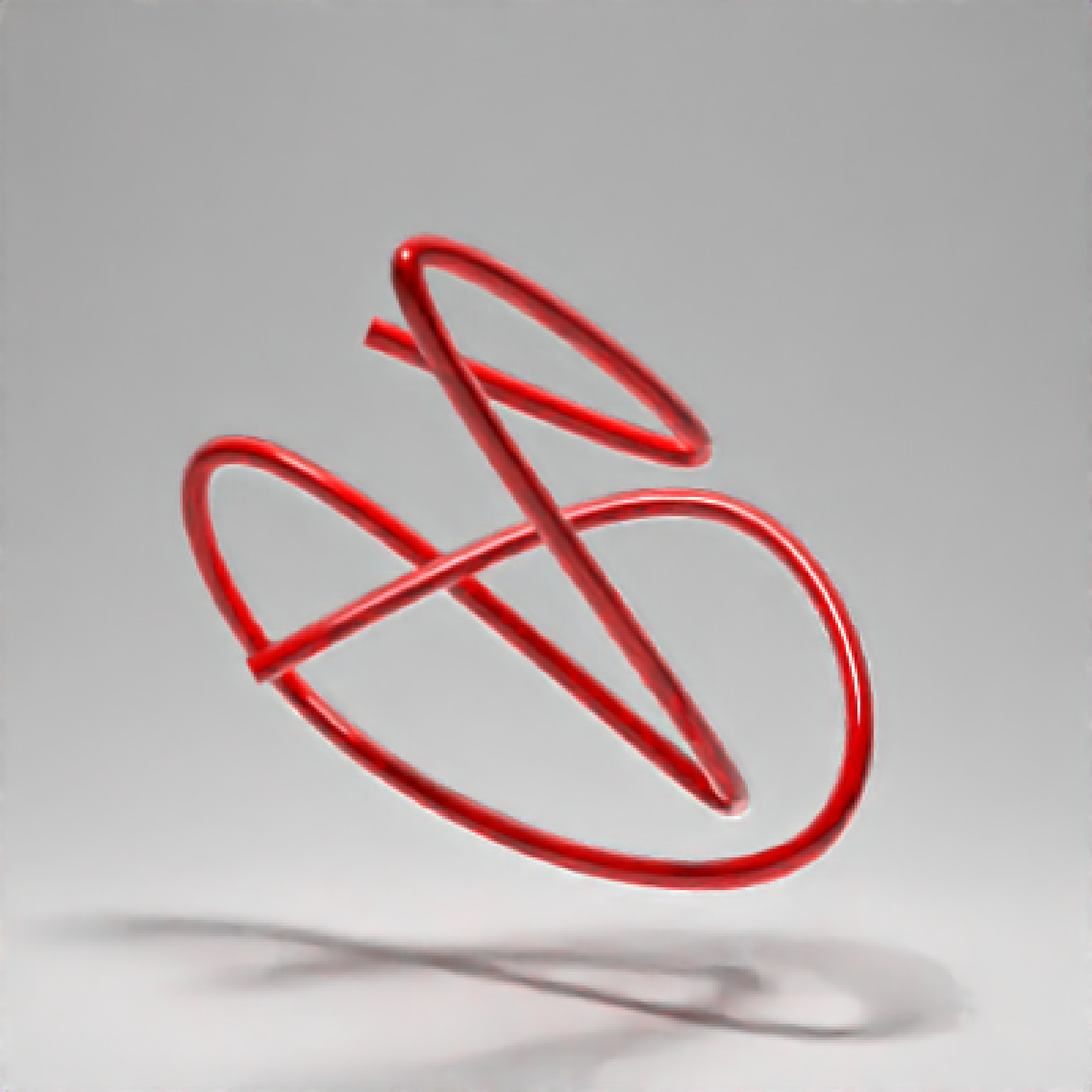if(run_documentation()) {
#We can ensure the curve is closed by setting closed = TRUE
generate_studio(depth=-1.5) %>%
add_object(path(points=random_mat, closed = TRUE, material=glossy(color="red"))) %>%
render_scene(samples=128, clamp_value=10)
}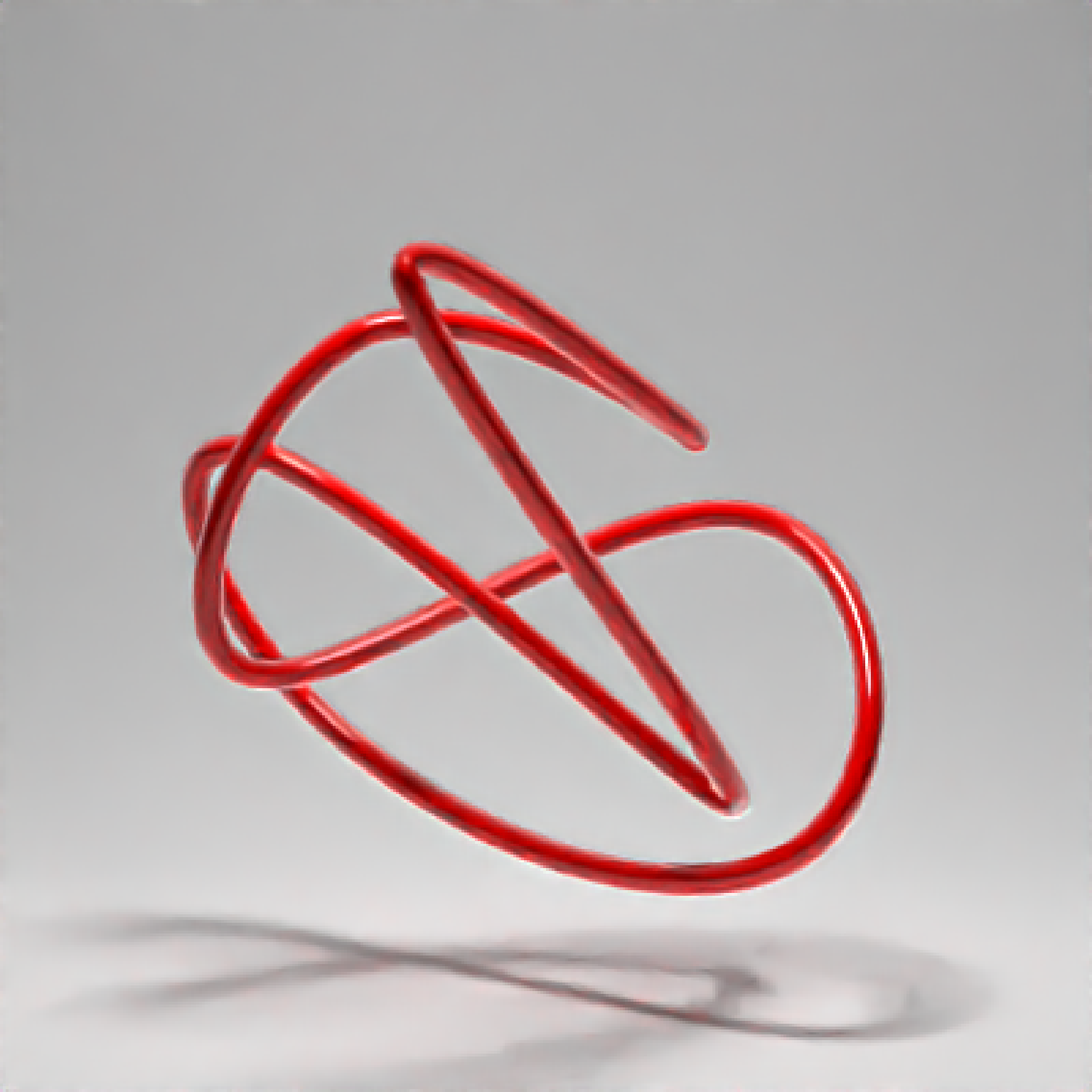if(run_documentation()) {
#Finally, let's render a pretzel to show how you can render just a subset of the curve:
pretzel = list(c(-0.8,-0.5,0.1),c(0,-0.2,-0.1),c(0,0.3,0.1),c(-0.5,0.5,0.1), c(-0.6,-0.5,-0.1),
c(0,-0.8,-0.1),
c(0.6,-0.5,-0.1),c(0.5,0.5,-0.1), c(0,0.3,-0.1),c(-0,-0.2,0.1), c(0.8,-0.5,0.1))

#Render the full pretzel:
generate_studio(depth = -1.1) %>%
add_object(path(pretzel, width=0.17,  material = glossy(color="#db5b00"))) %>%
render_scene(samples=128, clamp_value=10)
}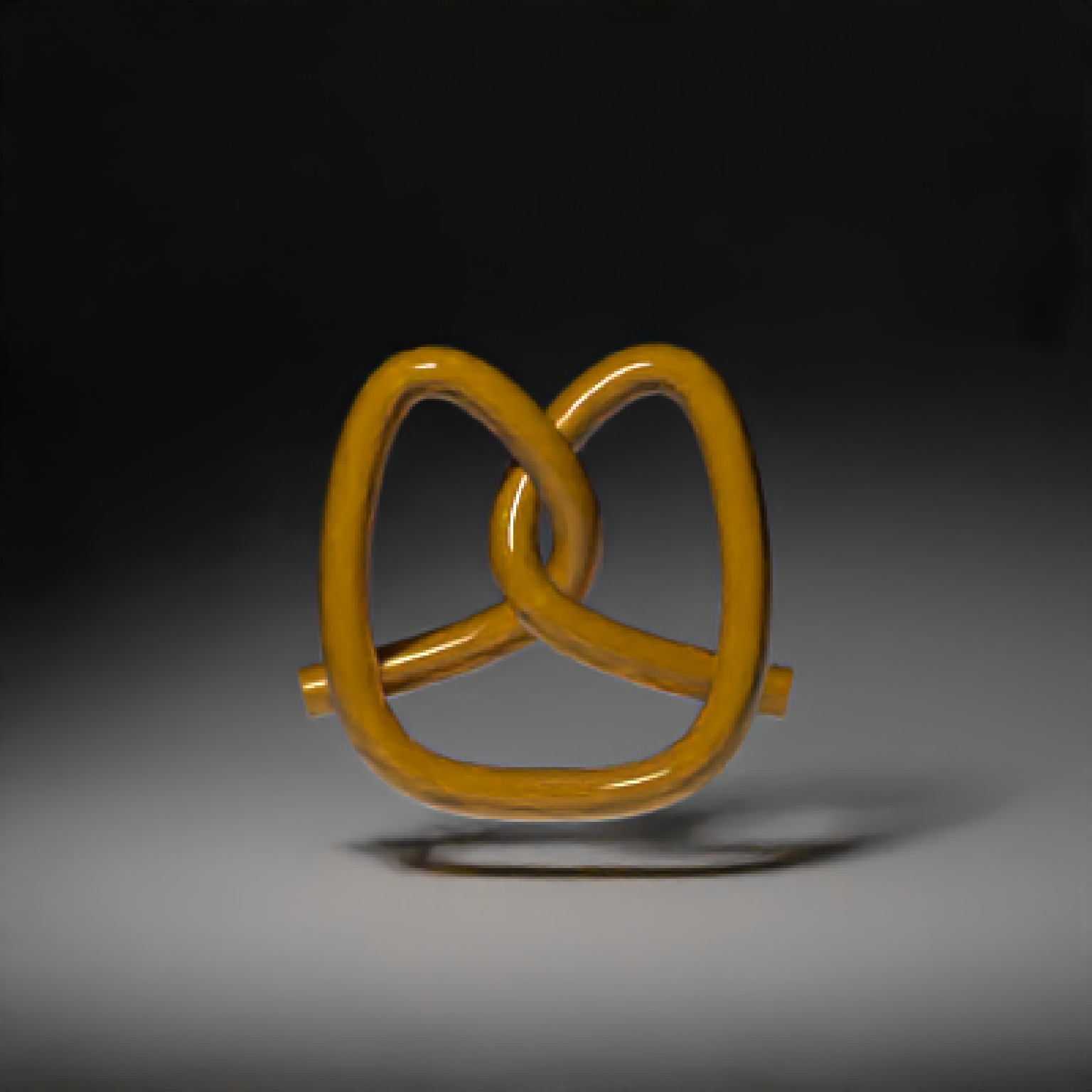if(run_documentation()) {
#Here, we'll render only the first third of the pretzel by setting u_max = 0.33
generate_studio(depth = -1.1) %>%
add_object(path(pretzel, width=0.17, u_max=0.33, material = glossy(color="#db5b00"))) %>%
render_scene(samples=128, clamp_value=10)
}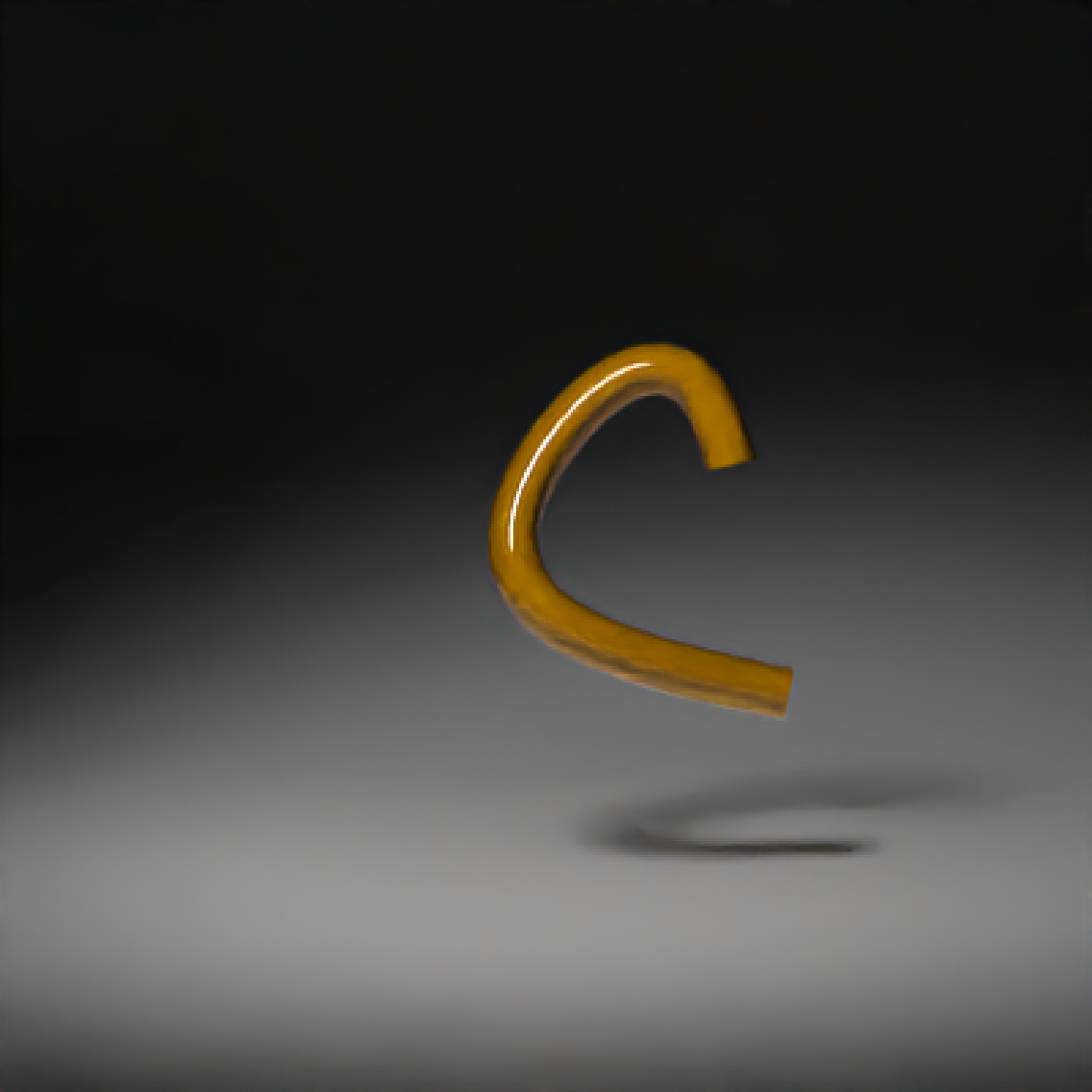if(run_documentation()) {
#Here's the last third, by setting u_min = 0.66
generate_studio(depth = -1.1) %>%
add_object(path(pretzel, width=0.17, u_min=0.66, material = glossy(color="#db5b00"))) %>%
render_scene(samples=128, clamp_value=10)
}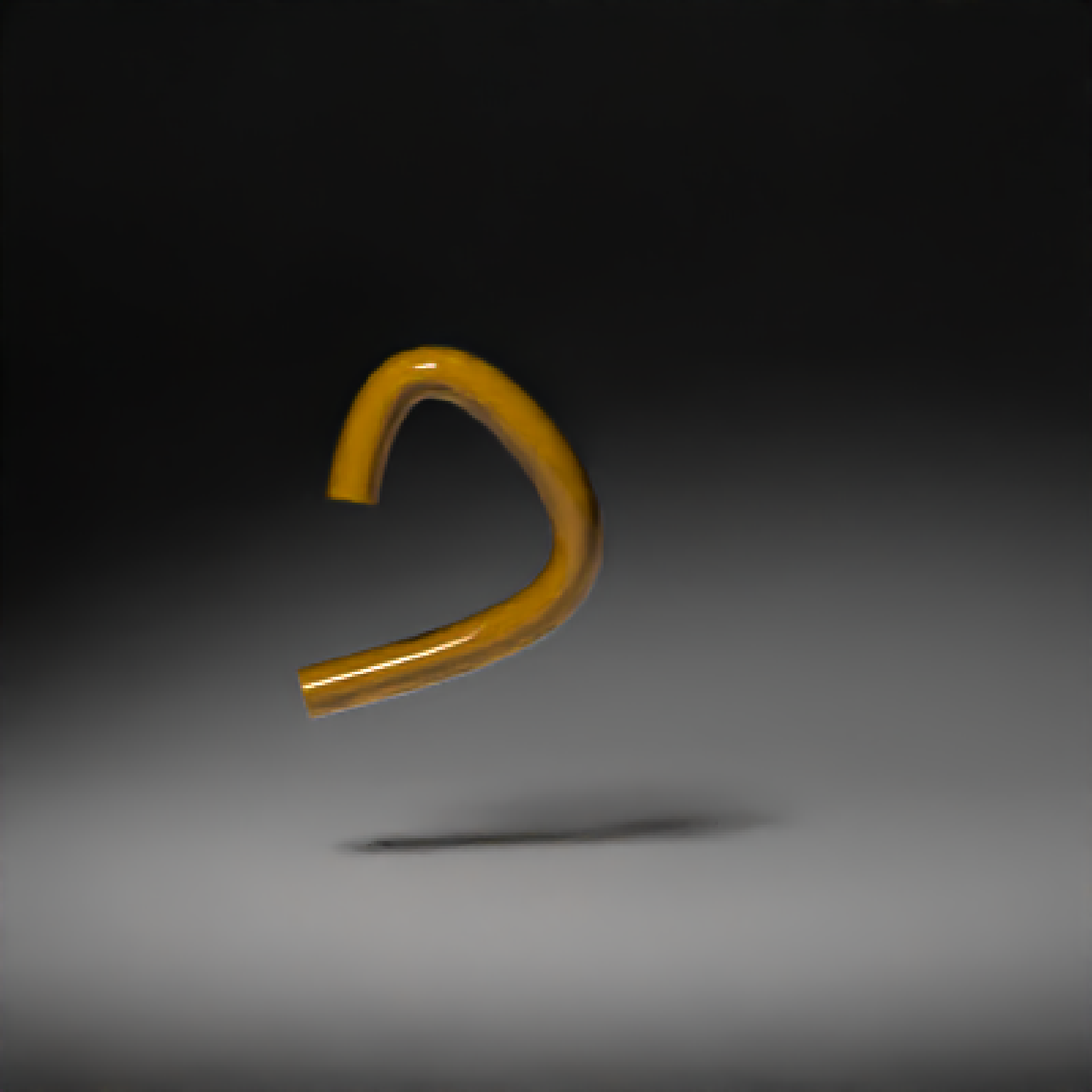if(run_documentation()) {
#Here's the full pretzel, decomposed into thirds using the u_min and u_max coordinates
generate_studio(depth = -1.1) %>%
add_object(path(pretzel, width=0.17, u_max=0.33, x = -0.8, y =0.6,
material = glossy(color="#db5b00"))) %>%
add_object(path(pretzel, width=0.17, u_min=0.66, x = 0.8, y =0.6,
material = glossy(color="#db5b00"))) %>%